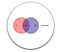# 8 Lines to Derive Naive Bayes Machine Learning Classifier

## In this article we try to derive the Naive Bayes algorithm from scratch in simple and easy language

TL:DR Skip to last section for 8 lines of straightforward derivation w/o explanation

I really believe in the philosophy that what you can’t create, you can’t understand clearly. While going through Machine learning algorithms, I came across Naive Bayes classifier. It made me curious to understand the logic behind the famous formula everyone was using and how it came into existence. This article tries to share my learning experience from scratch and in simple words. I am sure there are many curious minds out there and this article will surely help them.An example sample space for events A and b present in Universe U

Assume we have above Venn diagram, as a refresher following statements can be derived from it:

~where |A| or |B| means total elements in A or B . |U| means total elements in Universe

~See below image: P(A|B) means probability of A in the context of B, called as A given B. |AB| means |AB|

If we divide numerator and denominator by |U| i.e total elements in universe, we can have the rule generalised like this:

P(A|B) = (|AB|/|U| )/ (|B|/|U|)= P(AB)/P(B) i.e

Now if we combine above two rules in Point 3, we would have :

# Multiple Events and Chain Rule

From rule 3, we know P(AB) = P(A|B) . P(B). Now extending the same rule for 3 events ABC by induction:

P(A|BC) = P(ABC)/P(BC) OR P(ABC) = P(A|BC).P(BC)

and further applying the same rule to P(BC), we get

P(ABC) = P(A|BC).P(B|C).P(C)

OR

P(ABCD) = P(A|BCD).P(B|CD).P(C|D).P(D)

This is called Chain Rule.

Now as the number of events or attributes increase, the number of calculations we would require will also increase exponentially like:

Well, these are too many calculation! And can take forever to get an answer!

Question: Can we do something about it?

# Independent Events

Let’s assume that A and B are independent i.e if knowing whether A occurred gives no information about B (and vice versa).

For example: Consider the below question

What is the Probability of A given B i.e P(A|B) ?

If A and B are independent, then Probability of A given B is reduced only to probability of A, since both are independent and Given the context of B, A will not see any change. So P(A|B) is as good as P(A).

We also know P(A|B) = P(AB)/P(B) . Merging these rules we can say that for two independent events A and B:

P(AB) = P(A).P(B)

Now this is easy to calculate as compared to Rule 5. So we see if we have independent events, the probability is reduced to simple product of each event’s probability.

# Conditionally Independent Events

Now what if A and B are conditionally independent , Given the context C?

P(AB|C) = P(ABC)/ P(C) = P(A|BC) P(BC)/P(C)

P(AB|C) = P(A|BC).P(B|C)

So if A and B are independent P(A|BC) will be P(A|C) and P(B|AC) will be P(B|C), which gives us:

P(AB|C) = P(A|C). P(B|C)

OR generalising this, given conditionally independent events A1, A2, A3….An on C, we can have:

# Naive Bayes

Now lets use these rules of conditional independence in Bayes Theorem

In above figure, X1,X2….X5 are conditionally independent on Flu(F). So as per Bayes theorem:

P(F|X1,X2,X3,X4,X5) = P(F).P(X1,X2,X3,X4,X5|F)/P(X1,X2,X3,X4,X5)

Using Rule 6 and 7 here, we get:

= P(F).P(X1|F).P(X2|F)…P(X5|F) / P(X1)P(X2)…P(X5)

To generalise, we can conclude that if conditional independence is used with Bayes Theorem, we get something called as Naive Bayes. It is called Naive because of our naive assumption that events are independent on each other.

# Here is your 8 Lines Derivation Summary (in Order)

1. P(A) = |A| / |U| AND P(B) = |B| / |U|

2. P(A|B) = |AB| / |B|

3.P(A|B) = P(AB) / P(B) AND P(B|A) = P(AB) / P(A)

4. P(A|B) = P(A). P(B|A) / P(B) — Bayes Theorem

5. P(ABC) = P(A|BC).P(B|C).P(C) when A,B,C are dependent

6. P(ABC) = P(A).P(B).P(C) when A,B,C are independent

7. P(AB|C) = P(A|C). P(B|C) when A,B are conditionally independent on C

8. P(C|AB) = P(C).P(A|C).P(B|C)/P(A).P(B) — Naive Bayes via Points 4,6,7